• Excel公式函数汇总举例，详细描述了COUNTIF的各种用法。Excel
• [Excel常用函数] countif & countifs函数 玩转EXCEL系列-COUNTIF与COUNTIFS统计函数使用教程
[Excel常用函数] countif & countifs函数
玩转EXCEL系列-COUNTIF与COUNTIFS统计函数使用教程
展开全文• 在工作中,会经常遇到需要标记数据第几次出现的情况， 之前我用Excel时 公式是这样写的【=count($b$1:b1,b1)】然后下拉,但是数据量超过20万,就要等10分钟以上。 于是我用python的for循环测试20万数据，每想到还是很慢...
• 利用Excel COUNTIF函数对员工信息表中员工姓名排序.rar,本例展示了一份某企业的员工信息表，目前左表已经按员工工号排序，利用COUNTIF函数公式可以实现将员工姓名进行升序排列。Excel应用
• Excel统计函数COUNTIF()的常规用法介绍 COUNTIF函数常规用法一 基本简介作用 用来计算区域中满足给定条件的单元格的个数。语法讲解　COUNTIF(range,criteria) Range 为需要计算其中满足条件的单元格数目的...

Excel
统计函数COUNTIF()
的常规用法介绍

COUNTIF
函数常规用法一

基本简介作用

用来计算区域中满足给定条件的单元格的个数。

语法讲解　COUNTIF(range,criteria)

Range
为需要计算其中满足条件的单元格数目的单元格区域，即（范围）。

Criteria
为确定哪些单元格将被计算在内的条件，其形式可以为数字、表达式或文本，即（条件）。

例如：条件可以表示为 32
、"32"
、">32"
或 "apples"
。

说明：Microsoft Excel
提供其他函数，可用来基于条件分析数据。例如，若要计算基于一个文本字符串或某范围内的一个数值的总和，可使用 SUMIF
工作表函数。若要使公式返回两个基于条件的值之一，例如某指定销售量的销售红利，可使用 IF
工作表函数。

基本运用运用举例　　        1
、返回包含值12
的单元格数量

=COUNTIF
（DATA,12
）

2
、返回包含负值的单元格数量

=COUNTIF
（DATA
，"<0"
）

3
、返回不等于0
的单元格数量

=COUNTIF
（DATA
，"<>0"
）

4
、返回大于5
的单元格数量

=COUNTIF
（DATA
，">5"
）

5
、返回等于单元格A1
中内容的单元格数量

=COUNTIF
（DATA
，A1
）

6
、返回大于单元格A1
中内容的单元格数量

=COUNTIF
（DATA
，">''&A1
）

7
、返回包含文本内容的单元格数量

=COUNTIF
（DATA,''*''
）

8
、返回包含三个字符内容的单元格数量

=COUNTIF
（DATA
，''???''
）

9
、返回包含单词"GOOD"(
不分大小写)
内容的单元格数量

=COUNTIF
（DATA,''GOOD''
）

10
、返回在文本中任何位置包含单词"GOOD"
字符内容的单元格数量

=COUNTIF
（DATA,"*GOOD*"
）

11(1)
、返回包含以单词"AB"(
不分大小写)
开头内容的单元格数量

=COUNTIF
（DATA,"AB*"
）

11(2)
、返回包含以单词"AB"(
不分大小写)
结尾内容的单元格数量

=COUNTIF
（DATA,"*AB"
）

12
、返回包含当前日期的单元格数量

=COUNTIF
（DATA
，TODAY()
）

13
、返回大于平均值的单元格数量

=COUNTIF
（DATA
，">"&AVERAGE(DATA))

14
、返回平均值上面超过三个标准误差的值的单元格数量

=COUNTIF
（DATA,">"&AVERAGE(DATA)+STDEV(DATA)*3)

15
、返回包含值为3
或-3
的单元格数量

=COUNTIF
（DATA
，3
）+COUNIF
（DATA
，-3
）

16
、返回包含值;
逻辑值为TRUE
的单元格数量

=COUNTIF
（DATA
，TRUE
）

17
、统计区域中不为空的单元格个数（数值、文本、空格都算）——
（上述第3
条：文本也算不等于0
，空格不算）

=Countif(DATA,"<>")

18
、只统计文本单元格数量，不统计数值和空格——
（上述第7
条统计含空格）

=COUNTIF(DATA,"><")

具体举例

&#8203;

A

B

1

资料

资料

2

苹果

32

3

橘子

54

4

水蜜桃

75

5

苹果

86

公式
叙述

结果

=COUNTIF(A2:A5,"
苹果")
上述第一栏是苹果的储存格数目

2

=COUNTIF(B2:B5,">55")
以上第二栏中的数值大于 55
的储存格数目

2

扩展运用计算各种类型单元格的个数
真空单元格 =COUNTIF(data,"=")

真空+
假空单元格 =COUNTIF(data,"")

非真空单元格=COUNTIF(data,"<>")

文本型单元格=COUNTIF(data,"*")

判断条件表示不等于单个双引号“"”=COUNTIF(data,"<>""")

不连续区域求个数

三个区域中>=60=SUM(COUNTIF(INDIRECT({"a1:a10","b1:b10","c1:c10"}),">=60"))
多区域也可以扩展为多表求个数

两个条件求个数　　        >10
并且<=15 =SUM(COUNTIF(data,">"&{10,15})*{1,-1})

>=10
并且<15 =SUM(COUNTIF(data,">="&{10,15})*{1,-1})

>=10
并且<=15=SUM(COUNTIF(data,{">=10",">15"})*{1,-1})

>10
并且<15=SUM(COUNTIF(data,{">10",">=15"})*{1,-1})

用countif()
求区域内不重复值个数　　 {=sum(1/COUNTIF(A1:A10,A1:A10))} (
数组公式)

COUNTIF
函数常规用法二

一、求各种类型单元格的个数
(1)求真空单元格单个数:=COUNTIF(data,"=")
(2)真空+假空单元格个数:=COUNTIF(data,"")相当于countblank()函数
(3)非真空单元格个数:=COUNTIF(data,"<>")相当于counta()函数
(4)文本型单元格个数:=COUNTIF(data,"*")假空单元格也是文本型单元格
(5)区域内所有单元格个数:=COUNTIF(data,"<>""")
(6)逻辑值为TRUE的单元格数量：=COUNTIF(data,TRUE)
小说明：
EXCEL单元格内数据主要有以下几类:数值型,文本型,逻辑型,错误值型。其中时间类型也是一种特殊的数值。文本类型的数字是文本型。空单元格：指什么内容也没有的单元格，姑且称之为真空。假空单元格：指0字符的空文本，一般是由网上下载来的或公式得来的，姑且称之为假空。date指单元格区域，该参数不能是数组。

二、求><=
某个值的单元格个数
(1)大于50：=COUNTIF(data,">50")
(2)等于50：=COUNTIF(data,50)
(3)小于50：=COUNTIF(data,"<50")
(4)大于或等于50：=COUNTIF(data,">=50")
(5)小于或等于50：=COUNTIF(data,"<=50")
(6)大于E5单元格的值：=COUNTIF(data,">"&$E$5)
(7)等于E5单元格的值：=COUNTIF(data,$E$5)
(8)小于E5单元格的值：=COUNTIF(data,"<"&$E$5)
(9)大于或等于E5单元格的值：=COUNTIF(data,">="&$E$5)
(10)小于或等于E5单元格的值：=COUNTIF(data,"<="&$E$5)

三、等于或包含某N
个特定字符的单元格个数
(1)两个字符：=COUNTIF(data,"??")
(2)两个字符并且第2个是B:=COUNTIF(data,"?B")
(3)包含B:=COUNTIF(data,"*B*")
(4)第2个字符是B:=COUNTIF(data,"?B*")
(5)等于“你好”:=COUNTIF(data,"你好")
(6)包含D3单元格的内容:=COUNTIF(data,"*"&D3&"*")
(7)第2字是D3单元格的内容:=COUNTIF(data,"?"&D3&"*")
注：countif()函数对英文字母不区分大小写，通配符只对文本有效

四、两个条件求个数
(1)>10并且<=15=SUM(COUNTIF(data,">"&{10,15})*{1,-1})
(2)>=10并且<15=SUM(COUNTIF(data,">="&{10,15})*{1,-1})
(3)>=10并且<=15=SUM(COUNTIF(data,{">=10",">15"})*{1,-1})
(4)>10并且<15=SUM(COUNTIF(data,{">10",">=15"})*{1,-1})
注：一般多条件计数使用SUMPRODUCT函数，以上方法较少使用，仅供参考。
补充：三个区域计数：三个区域中=60

五、各种特殊统计要求的计算A1:A100
为存放数据的区域
（1）非空文本（仅包括可键入文本:=COUNTIF(A1:A100,">=!")
（2）所有非空文本=COUNTIF(A1:A100,">=!")+COUNTIF(A1:A100,">="&CHAR(1))-COUNTIF(A1:A100,">=")或{=SUM(COUNTIF(A1:A100,">="&{"!",""})*{1,-1})+COUNTIF(A1:A100,">="&CHAR(1))}即文本型-含" "(空格)数量
（3）全部可见单元格{=SUM(N(IF(ISERROR(A1:A100),1,SUBSTITUTE(A1:A100,"",""))<>""))}，即全部看上去不是空值的单元格(=全部记录-真空-空格)
（4）有效可见单元格=COUNTIF(A1:A100,">=!")+COUNTIF(A1:A100,">="&CHAR(1))-COUNTIF(A1:A100,">=")+COUNT(A1:A100)，即所有非空文+数字型数据之和
（5）全部不见单元格（真空＋空格＋空文本）=COUNTIF(A1:A100,"")+COUNTIF(A1:A100,">=!")+COUNTIF(A1:A100,">=")
（6）空格=COUNTIF(A1:A100,">=")-COUNTIF(A1:A100,">=!")
（7）空文本""=COUNTIF(A1:A100,"")-COUNTIF(A1:A100,"=")
（8）逻辑与错误=COUNTIF(A1:A100,"<>")-COUNTIF(A1:A100,"*")-COUNT(A1:A100)

展开全文• 我现在在做自动化报表，然后有一个问题一直卡着过不去，具体是：有两张表，一张是源数据（图1），一张是需要判断的数据（Sheet2），想实现"判断"列，"判断"列具体的公式是 “IFERROR(IF(OR(RIGHT(B2,1)="F",COUNTIF...有问必答 python
• 统计函数countif 使用COUNTIF函数检查中奖号码 (Check Winning Numbers with COUNTIF Function) Today, we’ll see a little magic from the COUNTIF function – it can do the work of seven formulas in one!...

统计函数countif

Today, we’ll see a little magic from the COUNTIF function – it can do the work of seven formulas in one! We'll use it to check winning numbers in an imaginary lottery.
今天，我们将从COUNTIF函数中看到一点魔力-它可以将七个公式合二为一！ 我们将使用它来检查假想彩票中的中奖号码。
The COUNTIF function can check a range of cells, and see if it contains a specific value. For example, type your lucky number in cell D2, and use a COUNTIF formula to see if that number is found in the list of winning numbers in cells I2:N2.
COUNTIF函数可以检查单元格范围，并查看其是否包含特定值。 例如，在单元格D2中键入您的幸运数字，并使用COUNTIF公式查看该数字是否在单元格I2：N2中的中奖号码列表中找到。
=COUNTIF(I2:N2,D2)
= COUNTIF(I2：N2，D2)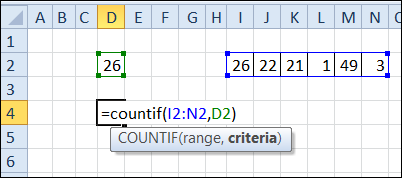The number, 26, is found once in the range I2:N2, so the COUNTIF result is 1. Your lucky number is a winner!
该数字26在I2：N2范围内被发现一次，因此COUNTIF结果为1。您的幸运数字是赢家！
检查多个数字 (Check Multiple Numbers)
What if we have multiple lucky numbers to check – not just one number? In the next screen shot, there are six numbers to check, entered in cells A2:F2. The winning numbers are still listed in cells I2:N2.
如果我们要检查多个幸运数字-而不仅仅是一个数字，该怎么办？ 在下一个屏幕快照中，在单元格A2：F2中输入了六个要检查的数字。 中奖号码仍列在单元格I2：N2中。
We could create a similar COUNTIF formula, to check each cell, to see if it is a winner. Here is the formula in B5 – the column references in I2:N2 are locked, so that reference won’t change when the formula is copied across to cell G5.
我们可以创建一个类似的COUNTIF公式来检查每个单元格，看看它是否是赢家。 这是B5中的公式– I2：N2中的列引用已锁定，因此将公式复制到单元格G5时，引用不会更改。
=COUNTIF($I2:$N2,D2)
= COUNTIF($I2：$ N2，D2)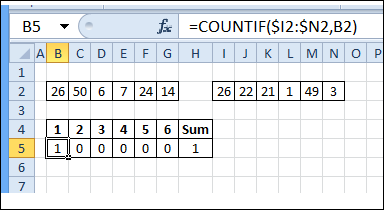In cell H5, use the SUM function to find the total number of cells that match the winning numbers.
在单元格H5中，使用SUM函数查找与获胜数字匹配的单元格总数。
=SUM(B5:G5)
= SUM(B5：G5)
That shows a total of 1, so only one of the six lucky numbers is a winner.
这表明总数为1，因此六个幸运数字中只有一个是获胜者。
一步获得总计数 (Get the Total Count in One Step)
Instead of creating 6 COUNTIF formulas, and a SUM, we could use an array-entered COUNTIF formula, to get the answer in one step.
代替创建6个COUNTIF公式和一个SUM，我们可以使用数组输入的COUNTIF公式来一步一步地得到答案。
In the next screen shot, the following formula is array-entered in cell B5 – press Ctrl + Shift + Enter, instead of just pressing Enter.
在下一个屏幕截图中，在单元格B5中输入了以下公式–按Ctrl + Shift + Enter ，而不是仅按Enter。
=SUM(COUNTIF(B2:G2,I2:N2))
= SUM(COUNTIF(B2：G2，I2：N2))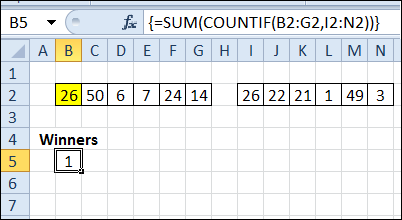If you change the lucky number in cell E2 to 22, instead of 7, the number of winners changes to 2, instead of 1. I’ve added conditional formatting in cells B2:G2, to colour the winning numbers yellow.
如果将单元格E2中的幸运数字更改为22，而不是7，则中奖者的数量更改为2，而不是1。我在单元格B2：G2中添加了条件格式 ，以将中奖数字涂成黄色。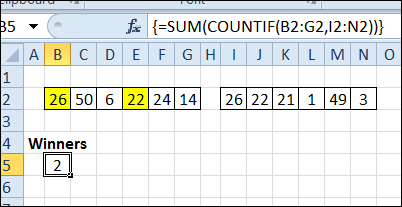With cells B2:G2 selected, the conditional formatting formula is the same as our earlier formula:
选择单元格B2：G2后，条件格式公式与我们之前的公式相同：
=COUNTIF($I2:$N2,B2)
= COUNTIF($I2：$ N2，B2)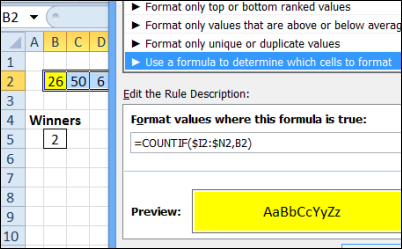检查失败的球队 (Check Losing Teams)
The same technique works with text too, and the range that’s being checked can be vertical, instead of horizontal.
相同的技术也适用于文本，所检查的范围可以是垂直的，而不是水平的。
In the next screen shot, 6 people have picked the teams that they think will win the four games being played today. When each game is finished, the losing team’s name is entered in column H.
在下一个屏幕快照中，有6个人选择了他们认为将赢得今天四场比赛的球队。 每场比赛结束后，输掉球队的名称会在H列中输入。
A SUM/COUNTIF array formula calculates how many losing teams each person picked. So far, 3 games are finished, and it’s looking good for Al. He hasn’t picked any losing teams yet!
SUM / COUNTIF数组公式可计算每个人选择的失败球队数。 到目前为止，已经完成了3场比赛，对于Al来说看起来不错。 他还没有挑选任何失败的球队！
Here is the formula array-entered in cell F2 (Ctrl + Shift + Enter), and copied down to cell F8.
这是在单元格F2中输入的公式数组(Ctrl + Shift + Enter)，并向下复制到单元格F8中。
=SUM(COUNTIF(B2:E2,$H$2:$H$5))
= SUM(COUNTIF(B2：E2，$H$ 2：$H$ 5))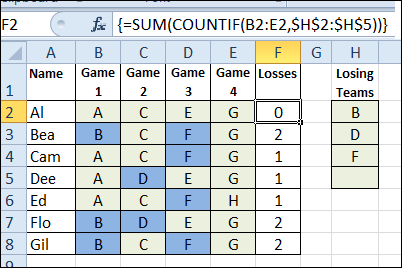Excel仪表板课程推荐 (Excel Dashboard Course Recommendation)
For a limited time, Mynda Treacy from My Online training Hub is opening her Excel Dashboard Course.
在有限的时间内，我的在线培训中心的Mynda Treacy正在开设她的Excel仪表板课程 。
The course is video based, delivered online and is available 24/7. You'll receive comprehensive workbooks and sample dashboards to keep, and there’s even an option to download the videos.
I've been through this course, and highly recommend it. The previous classes were very successful, and you can read the glowing reviews from the students, who loved all the techniques that they learned in the course, and are using them to impress their colleagues.
我已经完成了这门课程，并强烈推荐它 。 以前的课程非常成功，您可以阅读学生的热烈评论，他们喜欢他们在课程中学到的所有技术，并用它们给同事留下深刻的印象。
单击此处了解详细信息 ，阅读学生的评论，并观看“幕后花絮”视频，该视频向您展示您将获得的会员资格。

翻译自: https://contexturesblog.com/archives/2013/10/15/check-winning-numbers-with-countif-function/

统计函数countif

展开全文python 机器学习 java 人工智能 数据库
• excel countif 计算Excel数据COUNTIF COUNTA时出现问题 (Problems Counting Excel Data COUNTIF COUNTA) Last week, I ran into problems counting Excel data with COUNTIF, and it's Twitter's fault! Why did ...

excel countif

Last week, I ran into problems counting Excel data with COUNTIF, and it's Twitter's fault! Why did they do that? The COUNTA function can cause problems too, when it counts cells that look empty. Let's see how to fix both of those issues.
检查重复 (Check for Duplicates)
So, how did Twitter break my COUNTIF formula?
Every Thursday, I collect tweets for my weekly Excel Twitter post. The tweets are pasted into an Excel file, and a COUNTIF formula checks for duplicate content.
=COUNTIF(Used!C:C,H4)+COUNTIF(H:H,H4)
= COUNTIF(已使用！C：C，H4)+ COUNTIF(H：H，H4)
The result should be 1, unless there are duplicates
结果应为1，除非有重复项
Last Thursday, after years without problems, one row returned a #VALUE! error, instead of a number.
上周四，在没有问题的几年之后，一行返回了#VALUE！ 错误，而不是数字。

Recently, Twitter changed from a 140 character limit, to a 280 character limit. My workbook has a formula that checks the length of each tweet, and that one was 267 characters – the longest tweet that I've ever pasted into the workbook!
And yes, I have conditional formatting that highlights any tweet over 140 characters. Don't judge me.
是的，我有条件格式可以突出显示超过140个字符的任何推文。 不要判断我

COUNTIF个字符数限制 (COUNTIF Character Limit)
Unfortunately, Microsoft didn't raise its character limits, when Twitter increased theirs. That's what caused the error – COUNTIF/COUNTIFS can only check strings up to 255 characters. Other functions have the same limit.
不幸的是，当Twitter增加了字符限制时，Microsoft并未提高其字符限制。 这就是导致错误的原因– COUNTIF / COUNTIFS最多只能检查255个字符的字符串。 其他功能有相同的限制。
仅255个字符 (Only 255 Characters)
Here's another sample to show the problem. The 255 length works in row 4, but there is an error in row 5, which has one extra character – an X at the end.
这是另一个显示问题的示例。 255的长度在第4行有效，但在第5行有一个错误，该错误有一个额外的字符-结尾处是X。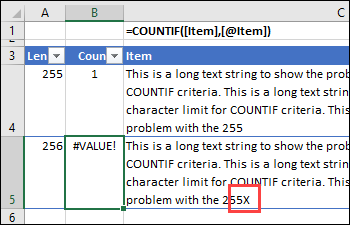To get a correct count, I used an old, reliable function --SUMPRODUCT, instead of COUNTIF. And since I was improving the formula, I created named ranges too, and wrapped it with an IF function.
为了获得正确的计数，我使用了一个可靠的旧函数--SUMPRODUCT，而不是COUNTIF。 由于我正在改进公式，因此我也创建了命名范围，并用IF函数将其包装起来。
=IF(H4="","",SUMPRODUCT(--(Tweets_Used=H4)) +SUMPRODUCT(--(Tweets_New=H4)))
= IF(H4 =“”，“”，SUMPRODUCT(-(Tweets_Used = H4))+ SUMPRODUCT(-(Tweets_New = H4)))
令人困惑的解决方法 (Confusing Workaround)
Later, I checked Microsoft's COUNTIF page, and it says you can get around the 255 limit, by joining two long strings with the concatenate operator (&). That suggestion did NOT work for me though - maybe I'm missing something:
后来，我检查了Microsoft的COUNTIF页面 ，它说，您可以通过使用连接运算符(＆)连接两个长字符串来绕过255个限制。 但是，该建议对我不起作用-也许我缺少了一些东西：
=COUNTIF(A2:A5,"long string"&"another long string") = COUNTIF(A2：A5，“长字符串”＆“另一个长字符串”)
Can you get that Microsoft formula to work?
您可以使该Microsoft公式起作用吗？
单击此处下载我的示例文件 ，并在我的网站上查看更多COUNTIF示例 。
COUNTA计算空单元格 (COUNTA Counts Empty Cells)
In other Excel count functions news, I've done a major update on my blog post about problems counting Excel data when cells look empty, but they aren't.
在其他Excel计数函数新闻中，我对博客文章进行了重大更新，内容涉及当单元格看起来为空时对Excel数据进行计数的问题 ，但事实并非如此。
In the screen shot below, data was copied from an Access database, and pasted into Excel. The COUNTA formula in cell C2 is counting those "blank" cells, even though they look empty.
在下面的屏幕快照中，从Access数据库复制了数据，并将其粘贴到Excel中。 单元格C2中的COUNTA公式正在计算那些“空白”单元格，即使它们看起来是空的。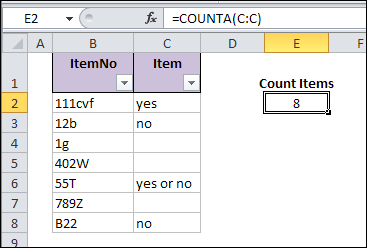其他原因 (Other Causes)
It's not just data from Access that creates these strange "blank" cells. They're also created if you convert formulas to values, and some of the formulas returned an empty string ("").
产生这些奇怪的“空白”单元的不只是来自Access的数据。 如果将公式转换为值，也会创建它们，并且某些公式返回空字符串(“”)。
This screen shot shows that type of formula, and when pasted as values, the empty string cells are counted.
此屏幕快照显示了该公式的类型，并在粘贴为值时对空字符串单元格进行计数。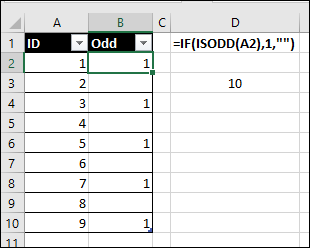请参阅隐藏的内容 (See Hidden Contents)
In the update, I added a tip that lets you see something in those "blank" cells.
在更新中，我添加了一个提示，使您可以在那些“空白”单元格中看到某些内容。
On the Excel Ribbon, click the File tab 在Excel功能区上，单击“文件”选项卡 At the left, click Option 在左侧，单击选项 In the Category list, click Advanced 在类别列表中，单击高级。 Scroll down to the end of the Advanced options, and look for the Lotus Compatibility section 向下滚动到“高级”选项的末尾，然后查找“ Lotus兼容性”部分。 Add a check mark to Transition Navigation Keys 在过渡导航键上添加复选标记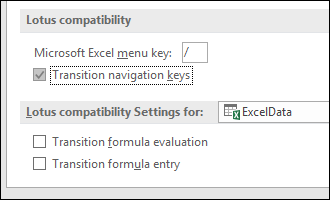After you turn this option on, click on a cell that looks blank, and check the Formula Bar. You should see an apostrophe there.
打开此选项后，单击看起来为空白的单元格，然后检查编辑栏。 您应该在那里看到一个撇号。
Remember to turn this option off later, when you've finished the troubleshooting.
完成故障排除后 ，请记住稍后关闭此选项 。
解决问题 (Fix the Problem)
My original solution was to use Find and Replace. The blanks were replaces with , and then the  were replaced with nothing. There are instructions to manually do those steps, and there's a macro too.
我最初的解决方案是使用“查找并替换”。 将空白替换为$$，然后将$$\$替换为空白。 有说明手动执行这些步骤，还有一个宏。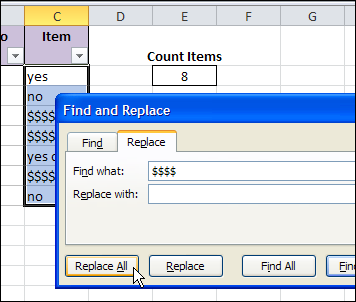The update has 2 new solutions, thanks to the people who posted their suggestions in the comments section.
此更新有2个新解决方案 ，这要归功于在评论部分发表了建议的人们。
How would you fix the problem?
您将如何解决该问题？

翻译自: https://contexturesblog.com/archives/2018/01/25/problems-counting-excel-data-countif-counta/

excel countif

展开全文java excel python 数据库 mysql
• countif（range，criteria） range：表示要计算其中非空单元格数目的区域 criteria：表示统计条件 eg：求得分大于等于80分小于90分的学生数
• Excel工作表“countif”条件计数函数、“countifs”多条件计数函数、“sumif”条件求和函数和“sumifs”多条件求和函数的应用举例
• 原作者问题：pandas如何简单实现excel中的countif函数？ 题主有如上所示的一个DataFrame，需要实现excel中countif函数，即统计每一行中除了指标之外的x1, x2, x3, x4中大于指标的个数。如第一行“4，5，5，3”中有3...pandas excel
• countif是Excel在工作中常用的一个函数，但是怎么样才能用好，避免条件一多就只能一个个数的苦闷吗？
• 使用Countif函数 Count函数 Countif函数 Countif函数计算数值区间 Countif函数超过15位字符时的错误 应用实例 在条件格式中使用Countif函数 在数据有效性中使用Countif函数 使用Countif函数 ...
• countif countif 在某个区域 统计 某个值的个数 在第一行到当前行区域 统计当前行value的个数 恰好就是分组求和 office官方文档 gif
• =COUNTIF(data,"=") (2) 真空+假空单元格个数: =COUNTIF(data,"") 相当于countblank()函数 (3) 非真空单元格个数: =COUNTIF(data,"<>") 相当于counta()函数 (4) 文本型单元格个数:...excel
• COUNTIF：对指定区域中符合指定条件的单元格计数的一个函数 该函数的语法规则如下： countif（range，criteria） 参数：range 要计算其中非空单元格数目的区域 参数：criteria 以数字、表达式或文本形式定义的...excel
• excel countif 这三个工作表功能执行相似的任务，但是它们之间存在关键区别。 COUNTIFS函数仅在Excel 2007和更高版本中可用。 COUNTIF（） COUNTIF函数用于“计数”给定范围内的一组设置条件的实例。 因此，您要在...python java mysql 数据库 excel
• 一、countif 函数的规则 1.count count：就是帮助计数的，得到的就是除去表头的数据 2.countif的基本操作 =COUNTIF(区域,条件)。 例如： 区域：点击要统计的这个列（或者行）。条件：点击要统计的代表单元格。 回车...excel
• countif()函数可以使用大于、大于等于、小于、小于等于等数学符号，对符合相应数据逻辑的单元格数量进行计数。 2、应用 1）返回包含负值的单元格数量。 =COUNTIF(range,"<0") 2）返回不等于0的单元格数量。 ...excel 区间统计
• 1、功能 ...countif()函数可以对一些指定的内容进行精确统计，如文字、数值、逻辑值等。 2、应用 1）求单元格内容为12的数量，range内的文本型"12"与数值12均会被统计。 公式：=COUNTIF(range,12)Excel
• excel很容易用countif函数来计数。。 但是我用pandas死活搞不出结果来。。 我的代码是： np.where(data[data.columns[1:]]> data['指标']).count(1) ---结果都是0 用: (data[data.columns[1:]]> data['指标'])....python
• Excel统计函数COUNTIF()的常规用法介绍 COUNTIF函数常规用法一 基本简介作用  用来计算区域中满足给定条件的单元格的个数。 语法讲解　COUNTIF(range,criteria)　 　Range 为需要计算其中满足...
• countif函数可实现对序号的数据进行排名。 2、应用 2.1 序号与排名 countif可以替代rank函数来进行排名统计。 美式排名： =COUNTIF(data,">"&B4)+1 不重复排名： =COUNTIF(data,">"&B4)+...excel
• 用到的函数：COUNTIF(要检查哪些区域? 要查找哪些内容?) 单元格A1的内容为：#NAME? 当单元格B7内输入 COUNTIF(A1,“) 后，得到的结果是1。注意：这里的“是中文状态下。 问题：中文状态下的“代表...sql
• 统计函数countif 快速更改Excel COUNTIF函数的结果 (Quickly Change Results for Excel COUNTIF Function)You're comfortable with Excel, and can change formulas on the fly, when necessary. If you're creating...excel python java 机器学习 人工智能
• 借助countif函数对数据表中的文本、数值进行判断并统计、计数。 2、应用 在下图1中，包括数值、文本型数值、文本等数据，下面就第一列的数据进行统计性应用。 图1 公式1： = COUNTIF(range,"<3") ...数据分析 excel 文本 数值
• ## SUMIF函数 countif函数

千次阅读 2017-03-01 13:10:25
countif函数 编辑 同义词countif一般指countif函数 Countif函数是Microsoft Excel中对指定区域中符合指定条件的单元格计数的一个函数，在WPS，Excel2003和Excel2007等版本中均可使用。 该函数的语法规则如下： ...
• countif函数去重计数 使用Excel COUNTIF函数对大于设置数量的单元格进行计数 (Count Cells Greater Than Set Amount With Excel COUNTIF Function) One of the tasks you have to do quite often in Excel is to ...python 机器学习 java excel 人工智能
• 举例： =COUNTIF(A2:A5,"China") =COUNTIF(A2:A5,A4) =COUNTIF(A2:A5,"<>"&B4)[不等于B4中的值] COUNTIF(A2:A5,"???na")[?代表占位符] countifs函数 countifs(区域,条件,[区域,条件]...) 区域和条件同时出现，至少......